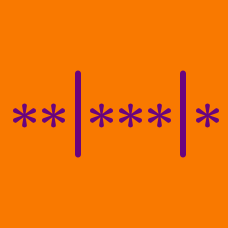Probability

# Distribution into Bins: Level 2 Challenges

There are 5 stars and 4 bars to be arranged in the 9 spaces pictured below. One possible arrangement is shown.How many possible arrangements are there?

Find the number of positive integer solutions of the equation

$x + y + z = 12.$

Find the number of 8-digit numbers the sum whose digits is 4.

How many (positive) seven digit integers are there that have a digit sum equal to $10$ and that are formed using only the digits $1$, $2$ and $3$?

There are 20 distinct people signed up for a raffle. There are 3 prizes that can be won: a 100 cm HDTV, a signed football jersey, and an envelope filled with gift cards. It is possible for a person to win more than one prize.

How many possible prize distributions are there?

×